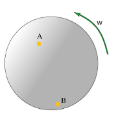# Problem: A merry-go-round is rotating at constant angular speed. Two children are riding the merry-go-round: Ana is riding at point A and Bobby is riding at point B. Part A Who moves with greater magnitude of tangential acceleration? Ana has the greater magnitude of tangential acceleration. Bobby has the greater magnitude of tangential acceleration. Both Ana and Bobby have the same magnitude of tangential acceleration. Part B Who has the greater magnitude of centripetal acceleration? Ana has the greater magnitude of centripetal acceleration. Bobby has the greater magnitude of centripetal acceleration. Both Ana and Bobby have the same magnitude of centripetal acceleration. Part C Who moves with greater magnitude of angular acceleration? Ana has the greater magnitude of angular acceleration. Bobby has the greater magnitude of angular acceleration. Both Ana and Bobby have the same magnitude of angular acceleration.

###### FREE Expert Solution

Part A

Tangential acceleration:

$\overline{){{\mathbf{a}}}_{{\mathbf{t}}}{\mathbf{=}}{\mathbf{r}}{\mathbf{\alpha }}{\mathbf{=}}{\mathbf{r}}\frac{\mathbf{∆}\mathbf{\omega }}{\mathbf{∆}\mathbf{t}}}$

Δω is zero for A and B.

79% (251 ratings)###### Problem Details

A merry-go-round is rotating at constant angular speed. Two children are riding the merry-go-round: Ana is riding at point A and Bobby is riding at point B.Part A Who moves with greater magnitude of tangential acceleration?

1. Ana has the greater magnitude of tangential acceleration.
2. Bobby has the greater magnitude of tangential acceleration.
3. Both Ana and Bobby have the same magnitude of tangential acceleration.

Part B Who has the greater magnitude of centripetal acceleration?

1. Ana has the greater magnitude of centripetal acceleration.
2. Bobby has the greater magnitude of centripetal acceleration.
3. Both Ana and Bobby have the same magnitude of centripetal acceleration.

Part C Who moves with greater magnitude of angular acceleration?

1. Ana has the greater magnitude of angular acceleration.
2. Bobby has the greater magnitude of angular acceleration.
3. Both Ana and Bobby have the same magnitude of angular acceleration.